Algebraic theory of linear
differential operators and
introduction to geometry of jet spaces

Joseph KRASIL'SHCHIK
A program of the course at the 1st Italian Diffiety School
(Forino, July 17  - August 1, 1998)
and at the 1st Russian Diffiety School
(Pereslavl-Zalessky, January 26 - February 5, 1998)

If you have difficulties with HTML,
kindly use TeX, DVI or PS-files
1.
Rings. Rings with unit. Associative rings. Commutative rings. Fields. Examples.
2.
Homomorphisms of rings. Kernels and images. Epi-, mono-, and isomorphisms. Algebras. Examples.
3.
Ideals. Quotient rings. Prime and maximal ideals.
4.
Modules. Generators. Submodules and quotient modules. Direct sums. Examples.
5.
Homomorphisms of modules. Kernels and images. Epi-, mono-, and isomorphisms. Exact sequences. Compositions of homomorphisms. Commutative diagrams.
6.
Homomorphisms of modules over commutative algebras. Bimodule structure.
7.
Free and projective modules.
8.
Tensor products. The tensor, symmetric and skew-symmetric algebras of a module.
9.
Categories and functors. Natural transformations of functors. Representable functors and representative objects.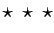10.
Vector fields on manifolds as derivations of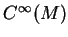. The Leibniz rule.
11.
Derivations of commutative algebras. Module and Lie algebra structures.
12.
Module-valued derivations. The modules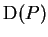. The commutator identity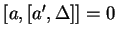. First order differential operators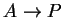and their description.
13.
The algebraic definition of linear differential operators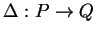. Equivalence of the algebraic and analytical definitions for the case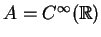.
14.
The bimodules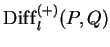. The tautological operators of order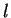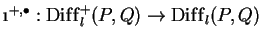and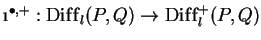. The bimodule embeddings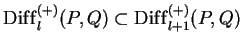, the module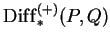. The bimodules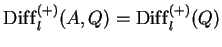.
15.
Composition of linear differential operators. The structure of an associative algebra in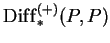.
16.
The exact sequences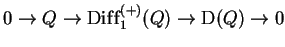and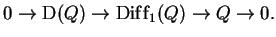The splitting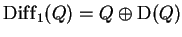.
17.
The operators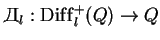, their universal property. The natural isomorphism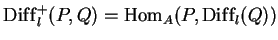. Representability of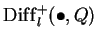.
18.
The universal composition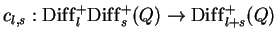as a natural transformation of functors. Associativity of the universal composition.19.
The submodules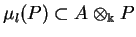. The jet modules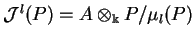. The operators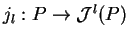.
20.
The universal property of operators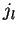. The natural isomorphism of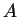-modules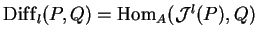. Representability of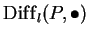.
21.
Functorial nature of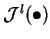. The exact sequences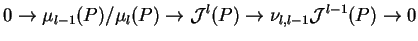22.
The module of infinite jets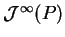.
23.
The universal cocomposition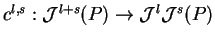as a natural transformation of functors. Coassociativity of the universal cocomposition.24.
The embedding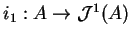,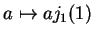,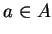. The module of 1-forms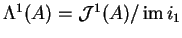. The de Rham operator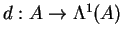,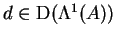. The splitting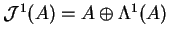.
25.
The universal property of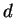. The natural isomorphism of-modules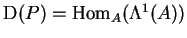. Representability of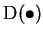.
26.
Description of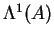by generators and relations.
27.
The modules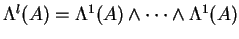, the module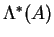. Wedge product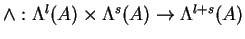in.
28.
The de Rham differential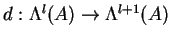, its properties. The de Rham complex and the de Rham cohomology.
29.
The Spencer jet complex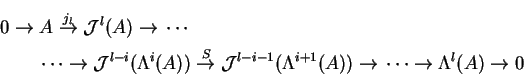30.
The modules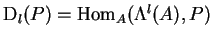. The functors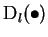.
31.
Elements of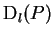as skew-symmetric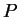-valued multiderivations.
32.
Wedge product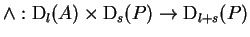, its properties. Structure of superalgebra in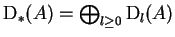.
33.
Inner product (contraction)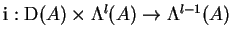, its properties.
34.
The formula35.
Action of derivations on differential forms (Lie derivative), its properties.
36.
The formula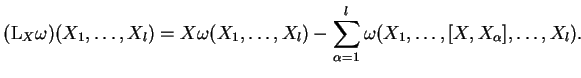37.
Smooth algebras.38.
Motivations to consider fiber bundles: how to extend algebras of observables? First examples: Cartesian products of manifolds, the Moebius band, tangent and cotangent bundles.
39.
Locally trivial fiber bundles. Morphisms. Sections. Subbundles.
40.
Pull-backs. Restriction of a bundle to a submanifold. Whitney products.
41.
Vector bundles. Morphisms. Kernels and images. Modules of sections, the functor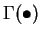.
42.
Direct sums and tensor products of vector bundles.
43.
Evaluation of-modules at points of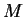. Isomorphism between the category of vector bundles overand the category of finitely generated-modules.44.
Example: evaluation ofat points of. Ghosts. The geometrization functor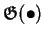and geometrical modules. Geometrization of algebraic Calculus over.
45.
Evaluation of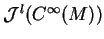at point of. Identification with Taylor series of order.
46.
General geometric definition of the manifolds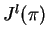. Points ofas classes of tangent sections. Jets of sections. Local coordinates.
47.
Fiber bundles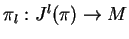and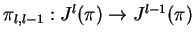. Vector bundle structure in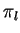. Fibers of the bundles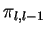.48.
Scalar nonlinear differential operators as functions on. General nonlinear differential operators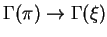.
49.
Nonlinear differential equations as submanifolds of. Solutions.
50.
How to diminish the order of a differential equation using the universal cocomposition?
51.
The Cartan distribution on, its maximal integral manifolds.
52.
Lie transformations and Lie fields. Symmetries.
53.
Passing to infinity.

References

1. M. F. Atiyah and I. G. MacDonald, Introduction to commutative algebra, Addison--Wesley Publ. Comp., Reading, Massachusetts, 1969.